×#### Thank you for registering.

One of our academic counsellors will contact you within 1 working day.

Click to Chat

1800-1023-196

+91-120-4616500

CART 0

• 0

MY CART (5)

Use Coupon: CART20 and get 20% off on all online Study Material

ITEM
DETAILS
MRP
DISCOUNT
FINAL PRICE
Total Price: Rs.

There are no items in this cart.
Continue Shopping```Independent Events

Table of Content

What are Independent Events?

How do you know if events are independent?

Formula of Independent Events

Problems

Difference between Independent and Mutually exclusive( or disjoint ) events

What are Independent Events?Let S be a sample space associated with the given random experiment. Let A and B be any two event defined on the sample space S. If the occurrence of any one event does not depend on occurrence or non-occurrence of other event, then two events A and B are said to be independent.

that is, If P(A/B) = P(A/B’) = P(A) or

P(B/A) = P(B/A’) = P(B)

Then A and B are independent events.

Example

A coin is tossed and it comes up "Heads" three times ... what is the chance that the next toss will also be a "Head"?

The chance is simply ½ (or 0.5) just like ANY toss of the coin.

What happened in past will not affect the current toss and so each toss of a coin is a perfect isolated thing.

Some examples of independent events are:

Outcome is head after tossing a coin AND rolling a 3 on a single 6-sided die.

Choosing a marble from a jar AND landing on head after tossing a coin.

Choosing a 4 from a deck of cards, replacing it, AND then choosing an ace as the second card.

Rolling a 5 on a single 6-sided die, AND then rolling a 2 on a second roll of the die.

How do you know if events are independent?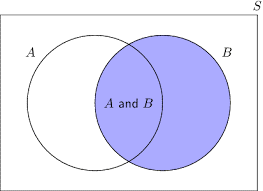If A and B are independent events then P (A∩B) = P(A).P(B)

In general, if A1, A2,…An are n independent events, then

P(A1 ∩ A2 ∩…. ∩ An) = P(A1).P(A2)…P(An)

Theorem:

If A and B are independent events then

(a) A and B’ are also independent

(b) A’  and B’  are also independent

Proof:

Since A and B are independent, therefore

P(A∩B) = P(A).P(B)

(a) P(A∩B’) = P(A) - P(A∩B)

= P(A) – P(A).P(B)

= P(A) [1- P(B)]

= P (A). P(B’)

Therefore, A and B’ are also independent

(b) P(A’ ∩ B’) = P(A U B)’             [By De Morgan’s Law]

= 1- P (A U B)

= 1- [P (A) +P (B) – P(A∩B)]

= 1- [P (A) +P (B) – P (A∩B)]

= 1- P (A) – P (B) + P (A).P (B)

= [1-P (A)] – P (B) [1-P (A)]

= [1-P (A)]. [1-P (B)]

= P (A’). P (B’)

Therefore, A’ and B’ are also independent

Formula of Independent Events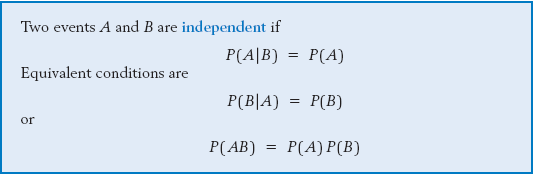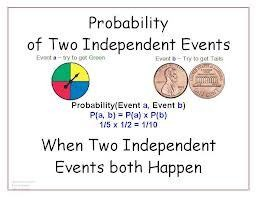Problems

Q1.  Two cards are drawn at random one after the other. Given that first card drawn is non-face red card, what is the probability that second card is face card, if the cards are drawn

i) Without Replacement? ii) With Replacement?

Sol. Let event A: first card is a non-face red card and event B : second card drawn is face card

P (A) = 20/52 = 5/13 and P(B) =12/52 = 3/13

Required probability = P (second card drawn is face card given that it is a red card)

= P(B/A)

i)  Without Replacement: Since first non-face red card is not replaced, therefore now we have 51 cards containing 12 face cards.

P(B/A) = 12/51 ≠ P(B). In this case A and B are not independent

ii)  With Replacement: Since first non-face red card is replaced, therefore now again we have 52 cards containing 12 face cards.

P (B/A) = 12/52 = 3/13 = P(B)

In this case A and B are independent

Q2.  A drawer contains a pair of socks each one with the following colours: blue, brown, red, white and black. Each pair is kept together folded in a matching set. You reach into the socks drawer and choose a pair of socks without looking. You replace this pair and then choose another pair of socks. What is the probability that you will choose the blue pair of socks both times?

Sol. P(blue) = 1/5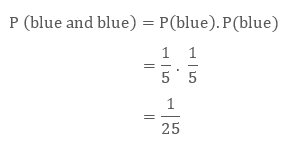Difference between Independent and Mutually exclusive( or disjoint ) events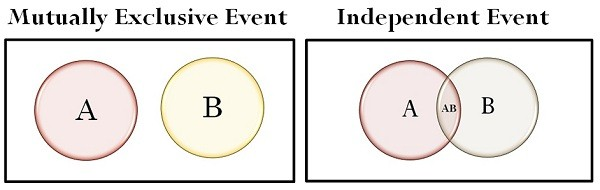If two events A and B are independent, then

P [A and B] = P [A]. P [B]

that is, the probability that both A and B occur is equal to the probability that A occurs times the probability that B occurs.

If A and B are mutually exclusive, then

P[A and B] = 0

that is, the probability that both A and B occur is zero.

Are all independent events disjoint events ( or mutually exclusive events )?

Clearly, if

A and B are nontrivial events (P [A] and P[B] are nonzero), that is,

P[A] > 0

P[B] >0,

then they cannot be both independent and mutually exclusive.

Example

Consider a fair coin and a fair six-sided die.

Let event A be obtaining heads, and event B be rolling a 6.  Here, the events A and B are independent, because the outcome of one does not affect the outcome of the other.  The probability that both A and B occur is

P[A and B] = P[A]P[B] = (1/2)(1/6) = 1/12.

Since this value is not zero, then events A and B cannot be mutually exclusive.

Basis for comparison

Mutually exclusive events

Independent events

Meaning

Two events are said to be mutually exclusive, when their occurrence is not simultaneous.

Two events are said to be independent, when the occurrence of one event cannot control the occurrence of other.

Influence

Occurrence of one event will result in the non-occurrence of the other.

Occurrence of one event will have no influence on the occurrence of the other.

Mathematical formula

P(A and B) = 0

P(A and B) = P(A) P(B)

Sets in Venn diagram

Does not overlap

Overlaps

To read more, Buy study materials of Probability comprising study notes, revision notes, video lectures, previous year solved questions etc. Also browse for more study materials on Mathematics here.

```### Course Features

• 731 Video Lectures
• Revision Notes
• Previous Year Papers
• Mind Map
• Study Planner
• NCERT Solutions
• Discussion Forum
• Test paper with Video Solution